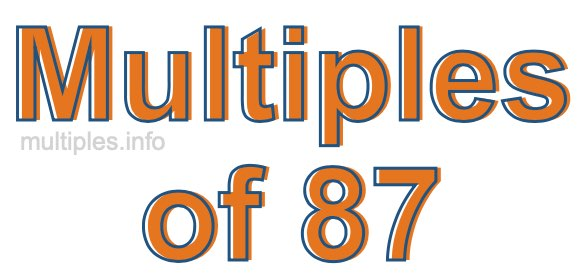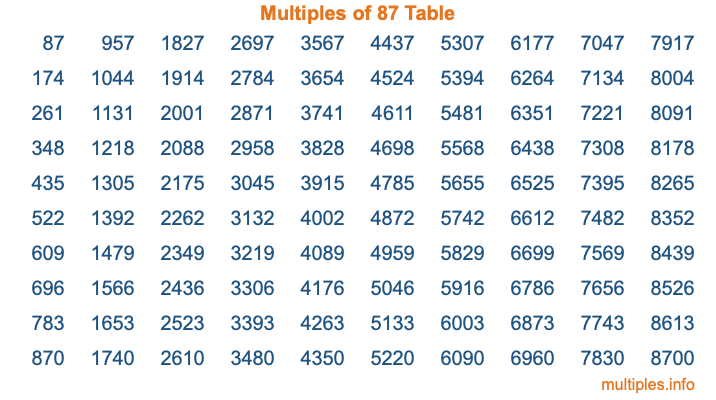Multiples of 87Welcome to the Multiples of 87 page. Here we will first teach you everything you will ever need to know about the multiples of 87, and then give you a study guide summary of everything we taught you to make sure you remember it all. Use this page to look up facts and learn information about the multiples of 87. This page will make you a multiples of eighty-seven expert!

Definition of Multiples of 87
Multiples of 87 are all the numbers that when divided by 87 equal an integer. Each of the multiples of 87 are called a multiple. A multiple of 87 is created by multiplying 87 by an integer.

Therefore, to create a list of multiples of 87, you start with 1 multiplied by 87, then 2 multiplied by 87, then 3 multiplied by 87, and so on for as long as you want. Thus, the list of the first five multiples of 87 is 87, 174, 261, 348, and 435. To see a larger list of multiples of 87, see the printable image of Multiples of 87 further down on this page. We also have a category where you can choose any nth multiple of 87.

Multiples of 87 Checker
The Multiples of 87 Checker below checks to see if any number of your choice is a multiple of 87. In other words, it checks to see if there is any number (integer) that when multiplied by 87 will equal your number. To do that, we divide your number by 87. If the the quotient is an integer, then your number is a multiple of 87.

Is  a multiple of 87?

Least Common Multiple of 87 and ...
A Least Common Multiple (LCM) is the lowest multiple that two or more numbers have in common. This is also called the smallest common multiple or lowest common multiple and is useful to know when you are adding our subtracting fractions. Enter one or more numbers below (87 is already entered) to find the LCM.

Check out our LCM Calculator if you need more details about the Least Common Multiple or if you need the LCM for different numbers for adding and subtraction fractions.

nth Multiple of 87
As we stated above, 87 is the first multiple of 87, 174 is the second multiple of 87, 261 is the third multiple of 87, and so on. Enter a number below to find the nth multiple of 87.

th multiple of 87

Multiples of 87 vs Factors of 87
87 is a multiple of 87 and a factor of 87, but that is where the similarities end. All postive multiples of 87 are 87 or greater than 87. All positive factors of 87 are 87 or less than 87.

Below is the beginning list of multiples of 87 and the factors of 87 so you can compare:

Multiples of 87: 87, 174, 261, 348, 435, etc.

Factors of 87: 1, 3, 29, 87

As you can see, the multiples of 87 are all the numbers that you can divide by 87 to get a whole number. The factors of 87, on the other hand, are all the whole numbers that you can multiply by another whole number to get 87.

It's also interesting to note that if a number (x) is a factor of 87, then 87 will also be a multiple of that number (x).

Multiples of 87 vs Divisors of 87
The divisors of 87 are all the integers that 87 can be divided by evenly. Below is a list of the divisors of 87.

Divisors of 87: 1, 3, 29, 87

The interesting thing to note here is that if you take any multiple of 87 and divide it by a divisor of 87, you will see that the quotient is an integer.

Multiples of 87 Table
Below is an image of the first 100 multiples of 87 in a table. The table is in chronological order, column by column. The first column has the first ten multiples of 87, the second column has the next ten multiples of 87, and so on.The Multiples of 87 Table is also referred to as the 87 Times Table or Times Table of 87. You are welcome to print out our table for your studies.

Negative Multiples of 87
Although not often discussed or needed in math, it is worth mentioning that you can make a list of negative multiples of 87 by multiplying 87 by -1, then by -2, then by -3, and so on, to get the following list of negative multiples of 87:

-87, -174, -261, -348, -435, etc.

Multiples of 87 Summary
Below is a summary of important Multiples of 87 facts that we have discussed on this page. To retain the knowledge on this page, we recommend that you read through the summary and explain to yourself or a study partner why they hold true.

There are an infinite number of multiples of 87.

A multiple of 87 divided by 87 will equal a whole number.

87 divided by a factor of 87 equals a divisor of 87.

The nth multiple of 87 is n times 87.

The largest factor of 87 is equal to the first positive multiple of 87.

87 is a multiple of every factor of 87.

87 is a multiple of 87.

A multiple of 87 divided by a divisor of 87 equals an integer.

87 divided by a divisor of 87 equals a factor of 87.

Any integer times 87 will equal a multiple of 87.

Multiples of a Number
Here you can get the multiples of another number, all with the same attention to detail as we did for multiples of 87 on this page.

Multiples of
Multiples of 88
Did you find our page about multiples of eighty-seven educational? Do you want more knowledge? Check out the multiples of the next number on our list!